# PSEB 9th Class Maths Solutions Chapter 8 Quadrilaterals Ex 8.1

Punjab State Board PSEB 9th Class Maths Book Solutions Chapter 8 Quadrilaterals Ex 8.1 Textbook Exercise Questions and Answers.

## PSEB Solutions for Class 9 Maths Chapter 8 Quadrilaterals Ex 8.1

Question 1.
The angles of a quadrilateral are in the ratio 3: 5 : 9: 13. Find all the angles of the quadrilateral.
Let, ABCD be a given quadrilateral.
∴ ∠A : ∠B : ∠C : ∠D = 3 : 5 : 9 : 13
Sum of ratios = 3 + 5 + 9 + 13 = 30
In quadrilateral ABCD, ∠A + ∠B + ∠C + ∠D = 360°
∴ ∠A = $$\frac{3}{30}$$ × 360° = 3 × 12 = 36°
∴ ∠B = $$\frac{5}{30}$$ × 360° = 5 × 12 = 60°
∴ ∠C = $$\frac{9}{30}$$ × 360° = 9 × 12 = 108°
∴ ∠D = $$\frac{13}{30}$$ × 360° = 13 × 12 = 156°
Thus the angles of the given quadrilateral are 36°, 60°, 108° and 156°.Question 2.
If the diagonals of a parallelogram are equal, then show that it is a rectangle.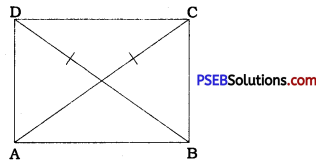In parallelogram ABCD, diagonals are equal.
∴ AC = BD.
In ∆ DAB and ∆ CBA.
DA = CB (Theorem 8.2)
AB = BA (Common)
DB = CA (Given)
∴ ∆ DAB ≅ ∆ CBA sss rule)
∴ ∠ DAB = ∠CBA (CPCT)
In parallelogram ABCD, AD || BC and AB is their transversal.
∴ ∠ DAB + ∠ CBA = 180°
(Interior angles on the same side of transversal)
Thus, in parallelogram ABCD, two angles ∠A and∠B are right angles. Hence, all the angles are right angle.
Hence, the parallelogram ABCD having equal diagonals is a rectangle.Question 3.
Show that if the diagonals of a quadrilateral bisect each other at right angles, then it is a rhombus.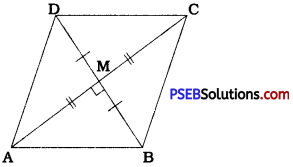In quadrilater ABCD. diagonals AC and BD bisect each other at M at right angles.
∴ AM = CM, BM = DM and
∠AMB = ∠CMB = ∠CMD = ∠AMD = 90°.
In ∆ AMB and ∆ CMB,
AM = CM
∠ AMB = ∠CMB
BM = BM (Common)
∴ ∆ AMB ≅ ∆ CMB (SAS rule)
∴ AB = CB (CPCT)
Similarly, proving ∆ BMC ≅ ∆ DMC and ∆ DMA ≅ ∆ BMA, we get BC = DC and DA = BA.
AB = BC CD = DA.
Therefore, quadrilateral ABCD is a rhombus.
Thus, if the diagonals of a quadrilateral bisect each other at right angles, then it is a rhombus.Question 4.
Show that the diagonals of a square are equal and bisect each other at right angles.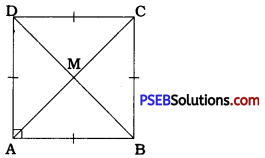ABCD is a square in which diagonals AC and BD intersect at M.
Every square is a parallelogram.
∴ AC and BD bisect each other. …………… (1)
In ∆ DAB and ∆ CBA,
DA = CB (Sides of a square)
∠ DAB = ∠ CBA (Right angles in a square)
AB = BA (Common)
∴ ∆ DAB ≅ ∆ CBA (SAS rule)
∴ BD = AC (CPCT) ……………….. (2)
Now, in ∆ AMB and ∆ CMB,
AM = CM (BD bisects AC at M).
BM = BM (Common)
AB = CB (Sides of a square)
∴ ∆ AMB ≅ ∆ CMB (SSS rule)
∴ ∠ AMB = ∠CMB (CPCT)
But, ∠ AMB and ∠ CMB form a linear pair.
∴ ∠ AMB + ∠ CMB = 180°
Hence, ∠AMB = ∠ CMB = 90° (3)
(1), (2) and (3) taken together proves that the diagonals of a square are equal and bisect each other at right angles.Question 5.
Show that if the diagonals of a quadrilateral are equal and bisect each other at right angles, then it is a square.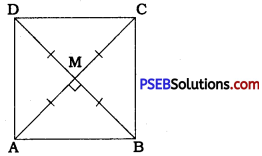In quadrilateral ABCD, diagonals AC and BD are equal and bisect each other at right angles.
∴ AC = BD,
MA = MC = MB = MD = $$\frac{1}{2}$$AC = $$\frac{1}{2}$$BD and
∠AMB = ∠CMB= ∠DMC = ∠DMA= 90°.
In ∆ AMB and ∆ CMB,
AM = CM
∠ AMB = ∠ CMB (Right angles)
BM = BM (Common)
∴ ∆ AMB ≅ ∆ CMB (SAS rule)
∴ AB = CB (CPCT)
Similarly, we can prove that BC = DC and
DA = BA.
AB = BC = CD = DA …………… (1)
Now, in ∆ DAB and ∆ CBA,
DA = C B
BD = AC (Given)
AB = BA (Common)
∴ ∆ DAB ≅ ∆ CBA (SSS rule)
∴ ∠DAB = ∠CBA (CPCT)
Thus, in quadrilateral ABCD, ∠A = ∠B.
Similarly, we can prove that ∠B = ∠C and ∠C = ∠D.
∠A = ∠B = ∠C = ∠D.
∠A + ∠B + ∠C + ∠D = 360°
∴ ∠A = ∠B = ∠C = ∠D = $$\frac{360^{\circ}}{4}$$ = 90° ……………… (2)
Thus, (1) and (2) taken together proves that in quadrilateral ABCD, all the sides are equal and all the angles are equal.
Therefore, quadrilateral ABCD is a square.
Thus, if the diagonals of a quadrilateral are equal and bisect each other at right angles, then it is a square.Question 6.
Diagonal AC of a parallelogram ABCD bisects ∠A (see the given figure). Show that (i) it bisects ∠C also, (ii) ABCD is a rhombus.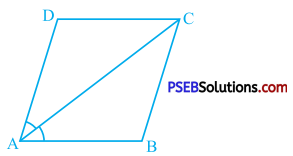Diagonal AC of parallelogram ABCD bisects ∠A.
∴ ∠DAC = ∠BAC …………… (1)
Now, ∠BAC and ∠DCA are alternate angles formed by transversal AC of AB || CD.
∴ ∠BAC = ∠DCA …………… (2)
Similarly, ∠DAC and ∠BCA are alternate angles formed by transversal AC of AD || BC.
∴ ∠DAC = ∠BCA ……………… (3)
From (1), (2) and (3),
∠DCA = ∠BCA.
But, ∠DCA + ∠BCA = ∠BCD (Adjacent angles)
∴ AC bisects ∠C also.
In parallelogram ABCD,
∠A = ∠C (Theorem 8.4)
∴ $$\frac{1}{2}$$∠A = $$\frac{1}{2}$$∠C
∴ ∠ DAC = ∠ DCA
∴ In ∆ DAC, DA = DC (Sides opposite to equal angles)
Moreover, in parallelogram ABCD,
AB = CD and BC = DA (Theorem 8.2)
∴ AB = BC = CD = DA
Thus. In parallelogram ABCD, all the sides are equal.
Hence, ABCD is a rhombus.Question 7.
ABCD is a rhombus. Show that diagonal AC bisects ∠A as well as ∠C and diagonal BD bisects ∠B as well as ∠D.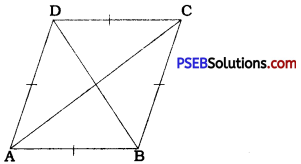ABCD is a rhombus
∴ AB || DC, BC || AD and AB = BC = CD = DA.
AB || DC and AC is their transversal.
∴ ∠CAB = ∠ACD (Alternate angles)
In, ∆ DAC, CD = DA
∴ ∠ CAB = ∠CAD = $$\frac{1}{2}$$ ∠DAB
This shows that AC bisects ∠A.
Again, BC || AD and AC is their transversal.
∴ ∠ BCA = ∠ DAC (Alternate angles)
In, ∆ DAC, DA = DC
∴ ∠ DAC = ∠ DCA
Then, ∠BCA = ∠DCA
But, ∠ BCA + ∠ DCA = ∠ DCB (Adjacent angles)
∴ ∠ BCA = ∠ DCA = $$\frac{1}{2}$$∠ DCB
This shows that AC bisects ∠C.
Thus, AC bisects ∠A as well as ∠C.
Similarly, taking BD as transversal of AB || DC, and BC || AD, it can be proved that BD bisects ∠B as well as ∠D.Question 8.
ABCD is a rectangle in which diagonal AC bisects ∠A as well as ∠C. Show that: (i) ABCD is a square. (ii) Diagonal BD bisects ∠B as well as ∠D.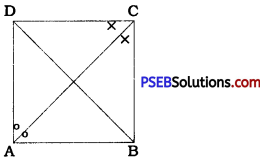In rectangle ABCD, AB = CD, BC = AD, AB || CD and BC || AD.
AC bisects ∠A as well as ∠C.
∴ ∠DAC = ∠BAC = $$\frac{1}{2}$$∠A and
∠ DCA = ∠ BCA = $$\frac{1}{2}$$∠C
Now, AB || CD and AC is their transversal.
∴ ∠ BAC = ∠ DCA (Alternate angles)
∴ ∠ DAC = ∠ DCA
Thus, in ∆ DAC, ∠DAC = ∠DCA
∴ AD = CD (Sides opposite to equal angles)
From this, we get AB = BC = CD = DA.
Also, in rectangle ABCD,
∠A = ∠B = ∠C = ∠D = 90°
Hence, ABCD is a square. …..Result (i)
In ∆ BCD, BC = CD
∴ ∠ CBD = ∠ CDB
Moreover, AB || CD and BD is their transversal.
∴ ∠ CDB = ∠ ABD (Alternate angles)
∴ ∠ CBD = ∠ ABD
Now, ∠ CBD + ∠ ABD = ∠ ABC
∴ ∠ CBD = ∠ ABD = $$\frac{1}{2}$$ ∠ ABC
Thus, BD bisects ∠B.
Similarly, diagonal BD bisects ∠ D.
Hence, diagonal BD bisects ∠B as well as ∠D …….. Result (ii)Question 9.
In parallelogram ABCD, two points P and Q are taken on diagonal BD such that DP = BQ (see the given figure). Show that:
(i) ∆ APD ≅ ∆ CQB
(ii) AP = CQ
(iii) ∆ AQB ≅ ∆ CPD
(iv) AQ = CP
(v) APCQ is a parallelogram.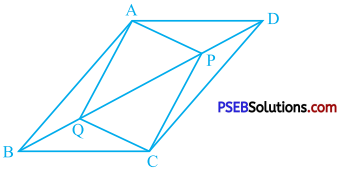ABCD is a parallelogram.
∴ AD || BC and BD is their transversal.
∴ ∠ADB = ∠CBD (Alternate angles)
∴ ∠ADP = ∠CBQ …………… (1)
Similarly, CD || BA and BD is their transversal.
∴ ∠ ABD = ∠ CDB (Alternate angles)
∴ ∠ABQ = ∠CDP ……………… (2)
In ∆ APD and ∆ CQB,
AD = CB (Opposite sides of a parallelogram)
∠ ADP = ∠ CBQ [by (1)]
DP = BQ (Given)
∴ ∆ APD ≅ ∆ CQB (SAS rule) ……. Result (i)
∴ AP = CQ (CPCT) …… Result (ii)
In ∆ AQB and ∆ CPD,
AB = CD (Opposite sides of a parallelogram)
∠ ABQ = ∠ CDP [by (2)]
BQ = DP (Given)
∴ ∆ AQB ≅ ∆ CPD (SAS rule) …….. Result (iii)
∴ AQ = CP (CPCT) ………….. Result (iv)
Now, in quadrilateral APCQ, AP = CQ and AQ = CP
Hence, by theorem 8.3, APCQ is a parallelogram. ………. Result (v)Question 10.
ABCD is a parallelogram and AP and Cg are perpendiculars from vertices A and C on diagonal BD (see the given figure). Show that
(i) ∆ APB ≅ ∆ CQD
(ii) AP = CQ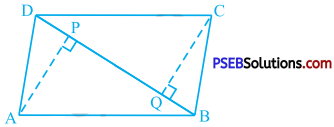In parallelogram ABCD, AB || CD and BD is their transversal.
∴ ∠ ABD = ∠ CDB (Alternate angles)
∴ ∠ABP = ∠CDQ ……………. (1)
Now, in ∆ APB and ∆ CQD,
AB = CD (Opposite sides of a parallelogram)
∠ ABP = ∠ CDQ [by (1)]
∠ APB = ∠ CQD (Right angles)
∆ APB ≅ ∆ CQD (AAS rule) ………… Result (i)
∴ AP = CQ (CPCT) ……….. Result (ii)Question 11.
In ∆ ABC and ∆ DBF, AB = DE, AB || DE, j BC = EF and BC || EF. Vertices A, B and C are joined to vertices D, E and F respectively (see the given figure). Show that:
(i) Quadrilateral ABED is a parallelogram
(ii) Quadrilateral BEFC is a parallelogram
(iv) Quadrilateral ACFD is a parallelogram
(v ) AC = DF
(vi) ∆ ABC ≅ ∆ DEF.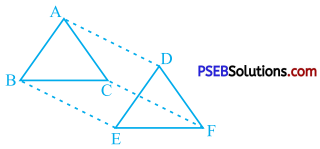In quadrilateral ∆ BED, AB = DE and AB || DE. Thus, in quadrilateral ABED, sides in one s pair of opposite sides are equal and parallel. Hence, by theorem 8.8, quadrilateral ABED is a parallelogram. …… Result (i)
Similarly, in quadrilateral BEFC, BC = EF and BC || EF.
Hence, by theorem 8.8, quadrilateral BEFC is a parallelogram. …………. Result (ii)
In parallelogram ABED, AD || BE and in parallelogram BEFC, BE || CE Thus, AD and CF both are parallel to BE.
In parallelogram ABED, AD = BE and in parallelogram BEFC, BE = CF.
∴ AD = CF ……… (2)
Taking (1) and (2) together, we get
AC and DF are opposite sides of parallelogram ACFD.
∴ AC = DF ………….. Result (v)
Now, in ∆ ABC and ∆ DEF,
AB = DE (Given)
BC = EF (Given)
AC = DF [by result (v)l
∴ ∆ ABC ≅ ∆ DEF (SSS rule) …… Result (vi)Question 12.
ABCD is a trapezium in which AB || CD and AD = BC (see the given figure). Show that:
(i) ∠A = ∠B
(ii) ∠C = ∠D
(iii) ∆ ABC ≅ ∆ BAD
(iv) diagonal AC = diagonal BD
[Hint: Extend AB and draw a line through C parallel to DA intersecting AB produced at E.)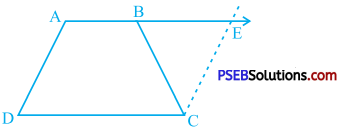AB is extended to E, and AB || CD.
∴ AE || CD
In quadrilateral ADCE, AE || CD and by consturction CE || DA.
∴ BC = CE
In ∆ BCE, BC = CE
∴ ∠CBE = ∠CEB
∴ ∠CBE = ∠CEA ………….. (1)
∴ ∠ DAE + ∠ CEA = 180° (Interior angles on the same side of transversal)
∴ ∠ DAE + ∠ CBE = 180° [by (1)]
∴ ∠ DAE = 180° – ∠ CBE …………… (2)
Moreover, ∠ ABC + ∠ CBE = 180° (Linear pair)
∴ ∠ ABC = 180° – ∠ CBE …………. (3)
From (2) and (3),
∠ DAE = ∠ ABC
∴ ∠A = ∠B ……… Result (i)
AB || CD and AD is their transversal.
∴ ∠A + ∠D = 180°
∴ ∠D = 180°- ∠A ………….. (4)
AB || CD and BC is their transversal.
∴ ∠B + ∠C = 180°
∴ ∠C = 180°- ∠B
∴ ∠C = 180° – ∠ A [by result (i)] ……… (5)
From (4) and (5),
∠C = ∠D …….. Result (ii)
Draw diagonals AC and BD.
In ∆ ABC and ∆ BAD,